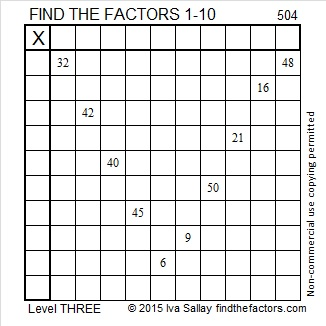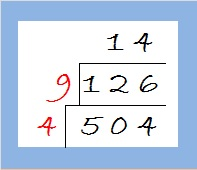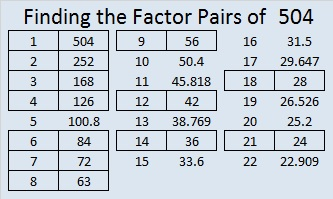# 504 and Level 3

Contents

### Today’s Puzzle:Print the puzzles or type the solution on this excel file: 10 Factors 2015-05-25

I’ve made a little cake to simplify √504:Just take the square root of everything on the outside of the cake and multiply them together: √504 = (√4)(√9)(√14) = (2 x 3)(√14) = 6√14 ≈ 22.449944, a pretty cool-looking approximation!

### Factors of 504:

• 504 is a composite number.
• Prime factorization: 504 = 2 x 2 x 2 x 3 x 3 x 7, which can be written 504 = (2^3) x (3^2) x 7
• The exponents in the prime factorization are 3, 2 and 1. Adding one to each and multiplying we get (3 + 1)(2 + 1)(1 + 1) = 4 x 3 x 2 = 24. Therefore 504 has exactly 24 factors.
• Factors of 504: 1, 2, 3, 4, 6, 7, 8, 9, 12, 14, 18, 21, 24, 28, 36, 42, 56, 63, 72, 84, 126, 168, 252, 504
• Factor pairs: 504 = 1 x 504, 2 x 252, 3 x 168, 4 x 126, 6 x 84, 7 x 72, 8 x 63, 9 x 56, 12 x 42, 14 x 36, 18 x 28 or 21 x 24
• Taking the factor pair with the largest square number factor, we get √504 = (√36)(√14) = 6√14 ≈ 22.449944### Sum-Difference Puzzle:

504 has twelve factor pairs. One of the factor pairs adds up to 65, and a different one subtracts to 65. If you can identify those factor pairs, then you can solve this puzzle!### A Puzzle about the Number 504:

The numbers 360, 420, 480, and 504 have something in common? The first three numbers are all multiples of 60, but 504 isn’t. 504 is the smallest number that isn’t divisible by 60 that has the same special something that those other three numbers have. Can you figure out what it is? Hint: It has something to do with the number 24.

### Factors for Today’s Puzzle:This site uses Akismet to reduce spam. Learn how your comment data is processed.